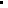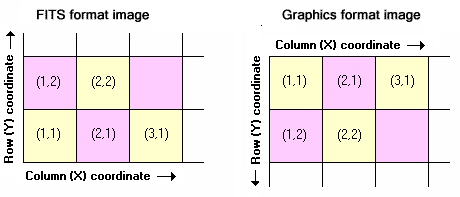Pixel Coordinate Definition

The location of a pixel, or array element, in the image is uniquely defined by its coordinates. THere are two ways to speciy the pixels coordinates: linear offset and axis coordinates.The linear offset is a sequential number ranging from 1 to the total number of pixels in the image.The Axis Coordinates define the pixel location as an array in multi-dimensional space. Each image axis has a length, in pixels, so that the image coordinates run between 1 and the length of the axis. THe total number of pixels in the image equals the product of the axis length for all image axes.Remember that the offset is 1-based, meaning that the first pixel of the image has nOffset=1.

Displaying Images

You must know how pixel coordinates are defined to answer the following types of questions: Where is column 42? Is column 42 actually 42 columns from the edge or is it 43 columns from the edge? is the edge at pixel 0 or at pixel 1? The FITS standard defines a protocol for this, which Mira adopts not just for FITS images, but for all image formats. As shown below, there are some differences in the way this works for different formats.Related Topics